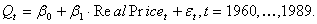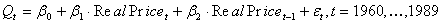# Introductory Econometrics

### Chapter 20: Autocorrelation

In this part of the book (Chapters 20 and 21), we discuss issues especially related to the study of economic time series. A time series is a sequence of observations on a variable over time. Macroeconomists generally work with time series (e.g., quarterly observations on GDPand monthly observations on the unemployment rate). Time series econometrics is a huge and complicated subject. Our goal is to introduce you to some of the main issues.

We concentrate in this book on static models. A static model deals with the contemporaneous relationship between a dependent variable and one or more independent variables.Asimple example would be a model that relates average cigarette consumption in a given year for a given state to the average real price of cigarettes in that year:In this model we assume that the price of cigarettes in a given year affects quantity demanded in that year.1In many cases, a static model does not adequately capture the relationship between the variables of interest. For example, cigarettes are addictive, and so quantity demanded this year might depend on prices last year. Capturing this idea in a model requires some additional notation and terminology. If we denote year t’s real price by RealPricet, then the previous year’s price is RealPricet-1. The latter quantity is called a one-period lag of RealPrice. We could then write down a distributed lag model:.

Although highly relevant to time series applications, distributed lag models are an advanced topic which we will not cover in this book.2

Let us return to the static model:As always, before we can proceed to draw inferences from regressions from sample data, we need a model of the data generating process.We will attempt to stick as close as possible to the classical econometric model. Thus, to keep things simple, in our discussion of static models we continue to assume that the X’s, the independent variables, are fixed in repeated samples. Although this assumption is pretty clearly false for most time series, for static models it does not do too much harm to pretend it is true. Chapter 21 points out how things change when one considers more realistic models for the data generating process.

Unfortunately, we cannot be so cavalier with another key assumption of the classical econometric model: the assertion that the error terms for each observation are independent of one another. In the case we are considering, the error term reflects omitted variables that influence the demand for cigarettes. For example, social attitudes toward cigarette smoking and the amount of cigarette advertising both probably affect the demand for cigarettes. Now social attitudes are fairly similar from one year to the next, though they may vary considerably over longer time periods. Thus, social attitudes in 1961 were probably similar to those in 1960, and those in 1989 were probably similar to those in 1988. If that is true and if social attitudes are an important component of the error term in our model of cigarette demand, the assumption of independent error terms across observations is violated.

These considerations apply quite generally. In most time series, it is plausible that the omitted variables change slowly over time. Thus, the influence of the omitted variable is similar from one time period to the next. Therefore, the error terms are correlated with one another. This violation of the classical econometric model is generally known as autocorrelation of the errors. As is the case with heteroskedasticity, OLS estimates remain unbiased, but the estimated SEs are biased.

For both heteroskedasticity and autocorrelation there are two approaches to dealing with the problem. You can either attempt to correct the bias in the estimated SE, by constructing a heteroskedasticity- or autocorrelation-robust estimated SE, or you can transform the original data and use generalized least squares (GLS) or feasible generalized least squares (FGLS). The advantage of the former method is that it is not necessary to know the exact nature of the heteroskedasticity or autocorrelation to come up with consistent estimates of the SE. The advantage of the latter method is that, if you know enough about the form of the heteroskedasticity or autocorrelation, the GLS or FGLS estimator has a smaller SE than OLS. In our discussion of heteroskedasticity we have chosen to emphasize the first method of dealing with the problem; this chapter emphasizes the latter method. These choices reflect the actual practice of empirical economists who have spent much more time trying to model the exact nature of the autocorrelation in their data sets than the heteroskedasticity.

In this chapter, we analyze autocorrelation in the errors and apply the results to the study of static time series models. In many ways our discussion of autocorrelation parallels that of heteroskedasticity. The chapter is organized in four main parts:

• Understanding Autocorrelation
• Consequences of Autocorrelation for the OLS estimator
• Diagnosing the Presence of Autocorrelation
• Correcting for Autocorrelation
Chapter 21 goes on to consider several topics that stem from the discussion of autocorrelation in static models: trends and seasonal adjustment, issues surrounding the data generation process (stationarity and weak dependence), forecasting, and lagged dependent variable models.

1We are implicitly assuming that changes in quantity demanded are due entirely to shifts in the supply curve. If this is not the case, a single equation model may be inappropriate.

2For a good treatment of distributed lag models, see Wooldridge (2003), pp. 326-329 and 601-607.

Excel Workbooks